# Algebra II : Multiplying and Dividing Exponents

## Example Questions

← Previous 1 3 4 5 6 7 8 9 18 19

### Example Question #3 : Simplifying Polynomials

Simplify.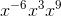Possible Answers: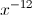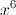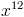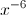Correct answer:Explanation:

Put the negative exponent on the bottom so that you have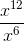which simplifies further to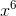.

### Example Question #1 : Multiplying And Dividing Exponents

Evaluate the following expression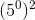Possible Answers: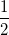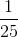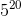Correct answer:Explanation: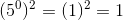We can also solve this problem using a different apporach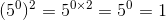Remember that any number raised to the 0th power equals 1

### Example Question #2 : Multiplying And Dividing Exponents

Simplify the following expression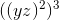Possible Answers: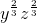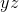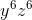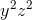Correct answer:Explanation: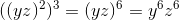Alternatively,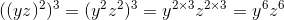### Example Question #3 : Multiplying And Dividing Exponents

Simplify the following expression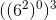Possible Answers: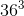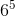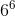Correct answer:Explanation: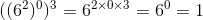Remember that any number raised to the 0th power equals 1

### Example Question #4 : Multiplying And Dividing Exponents

Evaluate the following expression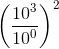Possible Answers: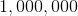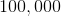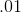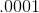Correct answer:Explanation: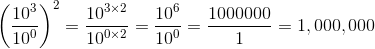Alternatively,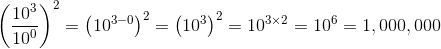### Example Question #5 : Multiplying And Dividing Exponents

Simplify: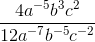Possible Answers: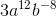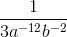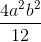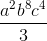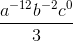Correct answer:Explanation:Step 1: Simplify the exponents using the division of exponents rule (subtract exponents in demoninator from exponents in numerator).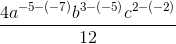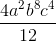Step 2: Reduce the fraction### Example Question #6 : Multiplying And Dividing Exponents

Simplify: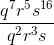Possible Answers: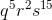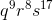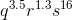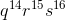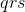Correct answer:Explanation:

Follow the division of exponents rule.  Subract the exponents in the denominator from the exponents in the numerator.

### Example Question #7 : Multiplying And Dividing Exponents

Rewrite using a single exponent.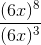Possible Answers: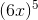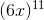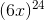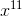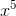Correct answer:Explanation:

Based on the property for dividing exponents: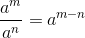In this problem, a is equal to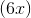, so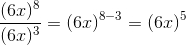### Example Question #8 : Multiplying And Dividing Exponents

Simplify: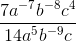Possible Answers: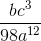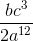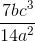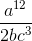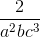Correct answer:Explanation:

Simplify:Step 1: Use the division of exponents rule, and subtract the exponents in the denominator from the exponents in the numerator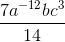Step 2: Move negative exponents in the numerator to the denominator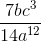Step 3: Simplify### Example Question #9 : Multiplying And Dividing Exponents

Simplify: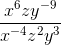Possible Answers: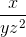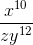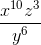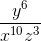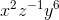Correct answer:Explanation:

Step 1: Use the division of exponents rule.  Subtract the exponents in the numerator from the exponents in the denominator.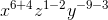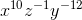Step 2: Represent the negative exponents as positive ones by moving them to the denominator: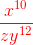← Previous 1 3 4 5 6 7 8 9 18 19

### All Algebra II Resources